# Qtr 1, Lesson 3: Rewriting Equations and Formulas

##By Stan Lisle

A lesson describing how to rewrite algebraic expressions with multiple variables and solve for any of the variables. Problems included to test the student's understanding.# One-step equations with multiplication and division

##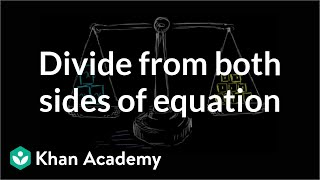By Khan Academy

Let's get a conceptual understanding of why one needs to divide both sides of an equation to solve for a variable.# One-step equations with multiplication and division

##By Khan Academy

Let's get a conceptual understanding of why one needs to divide both sides of an equation to solve for a variable.# Understanding negative number addition and subtraction with variables

##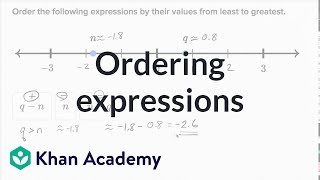By Khan Academy

Let's get some practice thinking about adding and subtracting variables representing positive and negative numbers on the number line.# Understanding negative number addition and subtraction with variables

##By Khan Academy

Let's get some practice thinking about adding and subtracting variables representing positive and negative numbers on the number line.# Understanding negative number addition and subtraction with variables

##By Khan Academy

Let's get some practice thinking about adding and subtracting variables representing positive and negative numbers on the number line.# Understanding negative number addition and subtraction with variables

##By Khan Academy

Let's get some practice thinking about adding and subtracting variables representing positive and negative numbers on the number line.# Understanding negative number addition and subtraction with variables

##By Khan Academy

Let's get some practice thinking about adding and subtracting variables representing positive and negative numbers on the number line.# Linear Equations in Two Variables

##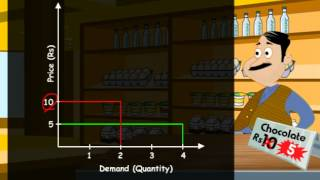By Meritnation

YouTube presents Linear Equations in Two Variables, an educational video resource on math.# Solving systems of equations in three variables

##By MathPlanetVideos

Solve the systems of equation in our example.# Equations with variables

##By MathPlanetVideos

Solve the following equation 8⋅x−x=21# Solving systems of equations in two variables

##By MathPlanetVideos

Solve the system of equations: {2x−4y=0 −4x+4y=−4# Equations with variables on both sides

##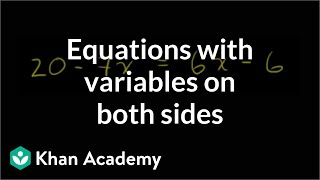By Khan Academy

Sal solves the equation 20 - 7x = 6x - 6.# Equations with variables on both sides

##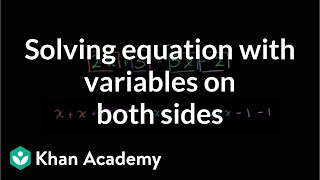By Khan Academy

Sal solves the equation 2x + 3 = 5x - 2.ï¿½ï¿½ï¿½ï¿½ï¿½ï¿½ï¿½ï¿½ï¿½ï¿½ï¿½ï¿½# 2.3 Quadratic Equations in One Variable Part 1

##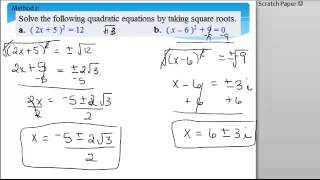By Tammy B

Solve quadratic equations in one variable.# Variables on both sides | Linear equations | Algebra I

##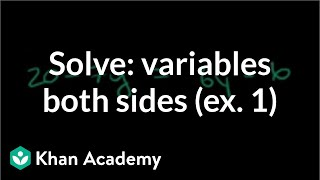By Khan Academy# Variables on both sides | Linear equations | Algebra I

##By Khan Academy

This video lecture series on Worked Examples in Algebra from Khan Academy includes Solving Equations, Solving Word Problems, Solving for a variable, Absolute Value and Number Lines, Patterns in Sequences, Functional Relationships, Domain and Range, Rate Problems, Linear Functions, Slope of a Line, X and Y intercepts, Equation of a Line, Parallel Lines, Perpendicular Lines, Solving Inequalities and more# Solving Quadratic Equations in one Variable by Factoring

##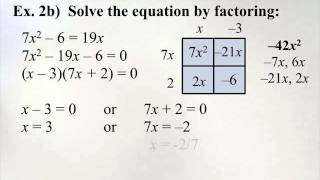By Victoriamiles

Solve quadratic equations in one variable.# Solve equations and simplify expressions

##By MathPlanetVideos

Solve the given equation 12(3b−b4a)=36# Different ways to solve equations

##By MathPlanetVideos

Solve the following expression 4x−7=x/3+4